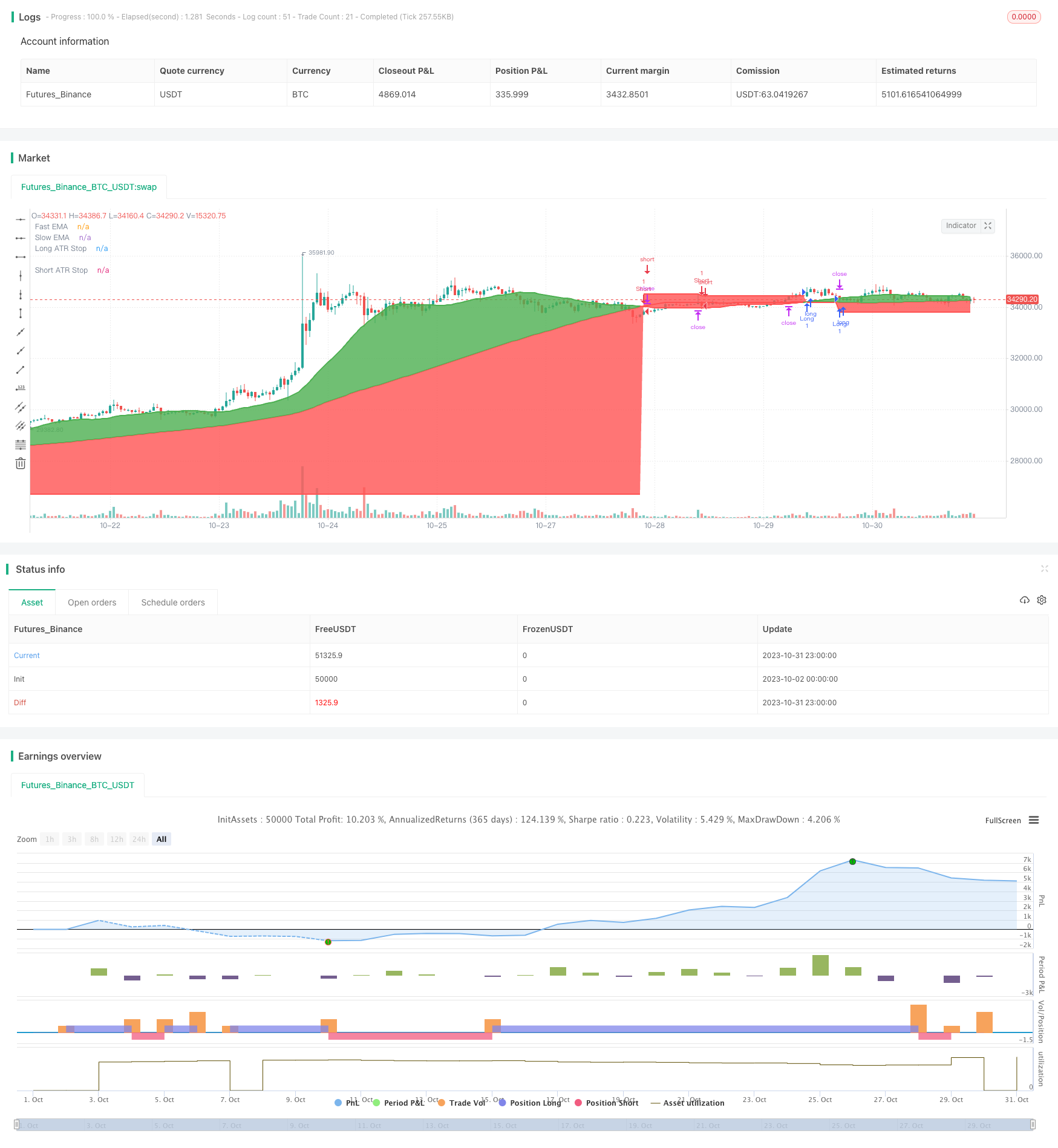# Dual Moving Average Crossover Trading Strategy

Author: ChaoZhang, Date: 2023-11-02 14:21:24
Tags:## Overview

This strategy uses the crossover of dual moving averages as trading signals, combined with ATR stops for trend following trading. The core idea is to go long when the fast moving average crosses above the slow moving average, and go short when crossing below, while using ATR to set stop loss levels for dynamically trailing stops.

## Strategy Logic

The strategy mainly uses two sets of moving averages to determine the trend direction. The fast moving average has a length of 25 days, and the slow moving average has a length of 100 days. A buy signal is generated when the fast MA crosses above the slow MA, and a sell signal is generated when crossing below.

To filter out some false signals, the strategy adds a crossover counter called crossCount. Signals are only triggered when the number of crosses for the fast MA in the lookback period (default 25 days) is less than maxNoCross (default 10).

In addition, the strategy has a confirmation mechanism, where the signal is also confirmed if the price re-enters between the two moving averages after the initial signal.

After entering positions, the strategy uses ATR to set stop loss levels. ATR measures the price fluctuation range over a certain period, and here its 14x is used as the stop distance. The stop level floats according to the price movement.

The strategy has the following advantages:

1. Using dual MA crosses with filtering, it can effectively capture strong trend movements while avoiding false signals.

2. The confirmation mechanism prevents being faked out by false breakouts.

3. The floating ATR stop loss helps maximise profits while limiting drawdowns.

4. It has few optimizable parameters and is easy to implement.

5. Applicable across markets including crypto and traditional assets.

6. Combines multiple technical indicators for robustness.

## Risk Analysis

The main risks of the strategy include:

1. Frequent MA crosses during range-bound periods can cause multiple losses.

2. Improper ATR parameter setting may lead to stops being too wide or too tight.

3. Large gaps can directly trigger stops.

4. Major news events causing huge volatility may also stop out positions.

5. Inadequate MA parameters could lead to missing trends or too many false signals.

6. Recent price action may render ATR stops outdated.

## Optimization Directions

The strategy can be further optimized in the following aspects:

1. Optimize the MA parameters to find better combinations, testing different periods and weighted averages.

2. Test different ATR periods to find better stop distances.

3. Add additional filters like volume spikes, volatility indicators to improve signal quality.

4. Incorporate trend metrics to avoid whipsaws in choppy markets.

5. Add machine learning algorithms to auto optimize parameters through backtesting.

6. Look for more confirmation on higher timeframes to avoid short term noise.

7. Implement staged profit taking rules to scale out of profitable positions.

## Summary

This strategy combines dual MA crosses, trend filtering, confirmation, and dynamic ATR stops for robust trend following. While there is room for improvement in optimization and risk control, the trading logic is simple and easy to replicate, making it a stable trend trading system.

```/*backtest
start: 2023-10-02 00:00:00
end: 2023-11-01 00:00:00
period: 1h
basePeriod: 15m
exchanges: [{"eid":"Futures_Binance","currency":"BTC_USDT"}]
*/

//@version=3

//MA's for basic signals, can experiment with these values

fastEMA = sma(close, 25)
slowEMA = sma(close, 100)

//Parameters for validation of position

lookback_value = 25
maxNoCross=10 //value used for maximum number of crosses on a certain MA to mitigate noise and maximise value from trending markets

//Amount of crosses on MA to filter out noise

ema25_crossover = (cross(close, fastEMA)) == true ? 1 : 0
ema25_crossover_sum = sum(ema25_crossover, lookback_value) ///potentially change lookback value to alter results
crossCount = (ema25_crossover_sum <= maxNoCross)

//Entries long

agrLong =  ((crossover(fastEMA, slowEMA)) and (crossCount == true)) ? true : false
consLong = ((close < fastEMA) and (close > slowEMA) and (fastEMA > slowEMA) and (crossCount == true)) ? true : false

//Entries short

agrShort =  ((crossunder(fastEMA, slowEMA)) and (crossCount == true)) ? true : false
consShort = ((close > fastEMA) and (close < slowEMA) and (fastEMA < slowEMA) and (crossCount == true)) ? true : false

//ATR

atrLkb = input(14, minval=1, title='ATR Stop Period')
atrRes = input("15",  title='ATR Resolution')
atr = request.security(syminfo.tickerid, atrRes, atr(atrLkb))

//Strategy

longCondition = ((agrLong or consLong) == true)
if (longCondition)
strategy.entry("Long", strategy.long)

shortCondition = ((agrShort or consShort) == true)
if (shortCondition)
strategy.entry("Short", strategy.short)

//Stop multiplier

stopMult = 4

//horizontal stoplosses

longStop = na
longStop :=  shortCondition ? na : longCondition and strategy.position_size <=0 ? close - (atr * stopMult) : longStop
shortStop = na
shortStop := longCondition ? na : shortCondition and strategy.position_size >=0 ? close + (atr * stopMult) : shortStop

//Strategy exit functions

strategy.exit("Long ATR Stop", "Long", stop=longStop)
strategy.exit("Short ATR Stop", "Short", stop=shortStop)

//Plots

redgreen = (fastEMA > slowEMA) ? green : red

p1 = plot(fastEMA, title="Fast EMA", color=redgreen, linewidth=2)
p2 = plot(slowEMA, title="Slow EMA", color=redgreen, linewidth=2)
fill(p1, p2, color=redgreen)

s1 = plot(longStop, style=linebr, color=red, linewidth=2,     title='Long ATR Stop')
s2 = plot(shortStop, style=linebr, color=red, linewidth=2,  title='Short ATR Stop')

fill(p2, s1, color=red, transp=95)
fill(p2, s2, color=red, transp=95)

```

More